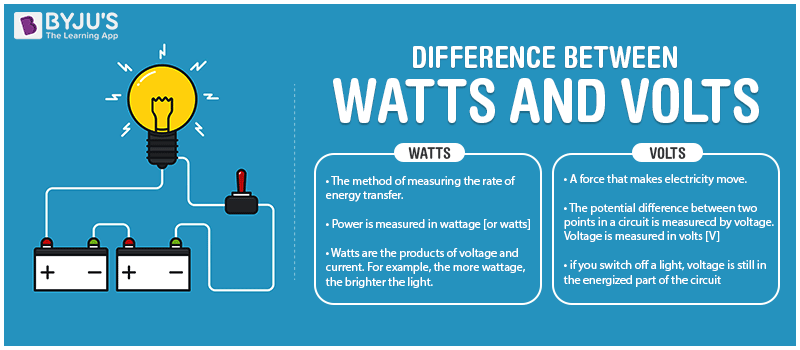# Difference Between Watts and Volts

The differences between watts and volts are given here in detail. Both volts and watts are related to each other. One watt is defined as the energy required by one ampere of current to flow through the potential difference of one volt. There are some specific differences between these two terms. These differences between watts and volts in tabular format will help the students to remember each point better and understand in-depth concepts effectively.Check the watts and volts differences in a tabular format below. Apart from knowing the differences, students can also understand their respective details in an easy and effective way. Check the volts and watts differences below.

Difference Between Watts and Volts:

 Sl. No. Differentiating Property Watts Volts 1 Basic Definition Watts is the SI unit of power (P). Volts is the SI unit of potential difference and EMF. 2 Explanation Watts specifies the rate at which energy is transferred. 1 volt is defined as the difference in electric potential across a wire when 1A current dissipates 1 watt of power across the wire. 3 Symbol W V 4 Formula One formula for watts is: W = Volts × Current One formula for volts is: V = Potential Energy/ Charge 5 Base Unit Kgm2s-3 Kgm2A-1s-3 6 Measuring Device Watts can be measured by using a Power meter. Volts can be measured by using a Voltmeter. 7 Measurement Details Watts gives a more realistic measurement for Power. Volts describe a small portion of voltage from the power source. 8 Measurement Difficulty Measuring Watts is difficult since it requires both voltage and current quantities. Measuring Volts is very easy when compared to Watts.

These were the main differences between volts and watts. It is important to be thorough with these differences to be able to attempt any related question in the physics exams also. These watts and volts differences and comparisons can also help to understand these two concepts in a better way.

Apart from the difference in volts and watts, students can also learn additional related topics. Check the articles given below to learn more similar concepts in a more effective and easy way.

Related Articles:

## This video will help you understand electricity in just 5 minutes!For more differences and formulas, keep visiting BYJU’S.

Test your knowledge on Watts and volts differences